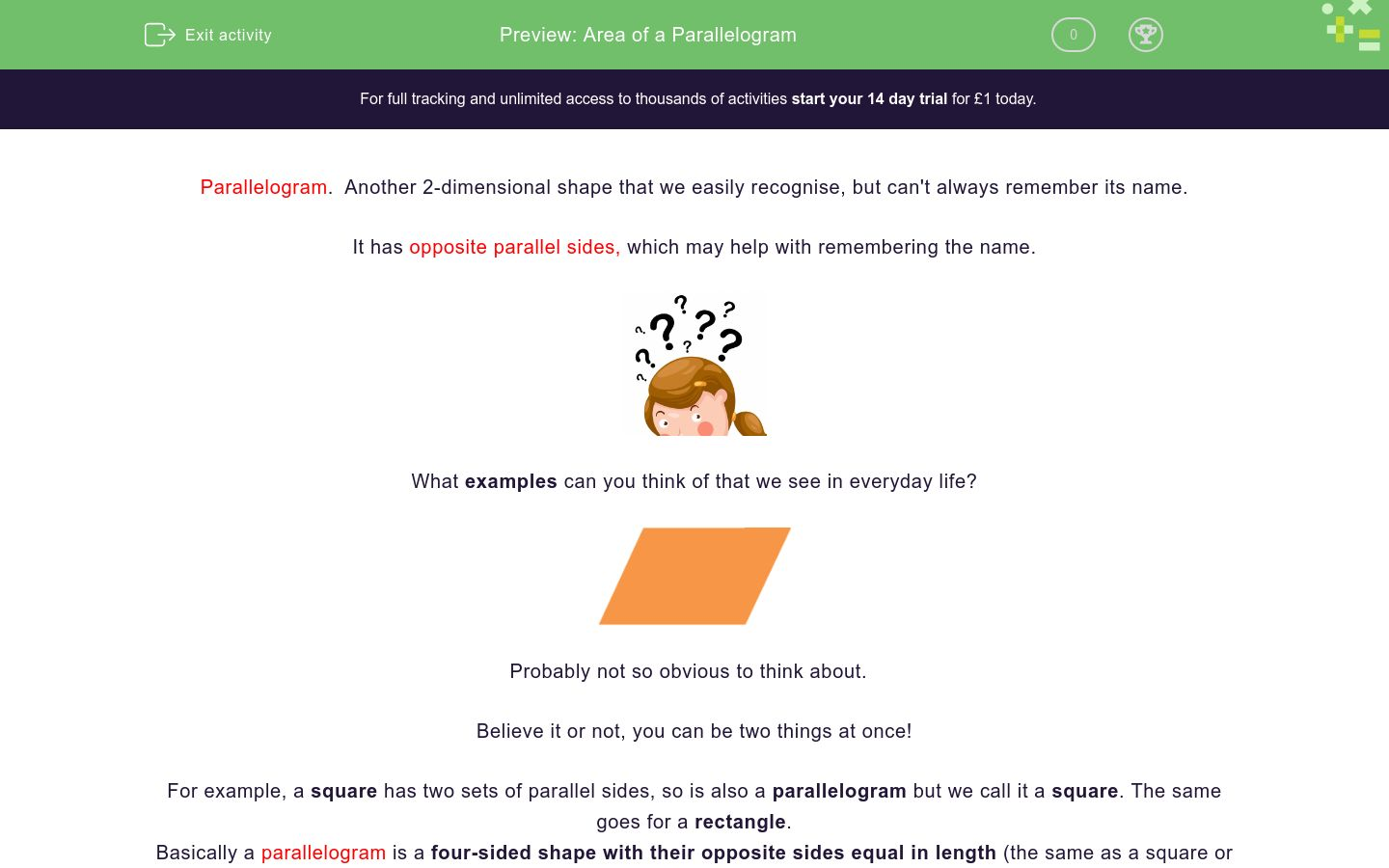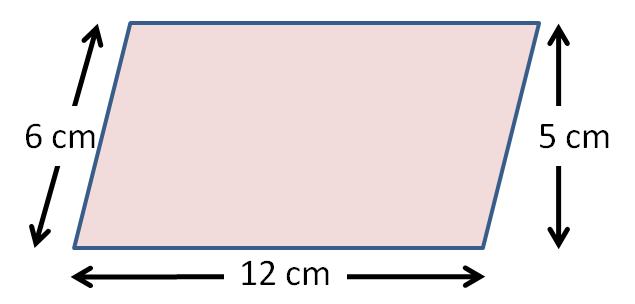# Area of a Parallelogram

In this worksheet students will learn the formula for finding the area of a parallelogram and be able to find areas and solve simple problems.Key stage:  KS 4

GCSE Subjects:   Maths

GCSE Boards:   AQA, Eduqas, Pearson Edexcel, OCR

Curriculum topic:   Geometry and Measures, Mensuration

Curriculum subtopic:   Mensuration and Calculation, Area Calculations

Difficulty level:### QUESTION 1 of 10

Parallelogram.  Another 2 dimensional shape that we easily recognise, but can't always remember its name.

I bet you call it a wonky rectangle when you can't remember its proper name.

It has opposite parallel sides, which may help with remembering the name.What examples can you think of that we see in everyday life?Probably not so obvious to think about.

Believe it or not you can be two things at once.

For example a square has two sets of parallel sides, so is also a parallelogram but we call it a square.The same goes for a rectangle.

Basically a parallelogram is a four sided shape with their opposite sides equal in length.(the same as a square or rectangle)Mathematicians can be awkward and make a four sided look different just for fun, so that's how we arrive at  parallelogram.

There are all sorts of reasons we may need to know the area of a parallelogram

Before we can do any of this there is one thing we need to know and that is  how to find the area of a parallelogram.

First of all we know that the sides of a parallelogram are not all the same length.

Two sides will have one length and the other two sides a different length.As with a lot of shape work there is a formula that you need to learn. So here goes

Area of a parallelogram =base x height

Yes, really that is all there is to it.

You may recognise that it is the same as the formula for the area of a square and a rectangle.

Find the area of the parallelogram above.

Area = base x height

Area = 15 cm x 3 cm = 45 cm² .

As we are working with area units are always squared. e.g cm² m²Look out.  You need to work with the vertical height not the slanted height.

12 x 5 = 60 cm&sup2;

What a bargain - one formula for three shapes.Find the area of this parallelogram.

14 cm²

36 cm²

60 cm²

64 cm²

Match these areas

## Column B

Parallelogram sides 4 cm and 3cm
12 cm²
Parallelogram sides 6 cm and 5 cm
62.05 cm²
Parallelogram sides 11 cm and 3 cm
20 mm²
Parallelogram sides 8.5 cm and 7.3 cm
30 cm²
Parallelogram sides 4cm and 5 cm
22.26 m²
Parallelogram sides 5.3 m x and 4.2 m
33 cm²Find the area  of this parallelogram.A farmer has a  parallelogram pen for his horses measuring  2.7m along one fence line and 212 cm along the other fence line.  What is the area of the floor of the barn?

5724 m²

5.624 m²

5.724 m²

5.64 m²What is the difference between a parallelogram with side lengths of 28 cm and 4 cm and a parallelogram of 37 by 5.5 cm?Two new rugs in the shape of a parallelogram have been bought for this families living room.

One rug measures  4 m by 2 m.  The other is 6m by 4m.  What is the total area of the rugs?

30 m²

24 cm²

32 cm²

18 cm²What is the area of these parallelograms?

 80 cm² 18 cm² 84 cm² 19 cm² don't know Parallelogram A; Parallelogram BThis mosaic is going to have parallelogram tiles placed around it.  The tiles are painted, Brown, beige and black.

The brown parallelograms have sides of  3 mm and 2 mm

The beige parallelograms have sides of  3 cm and 2.5 cm

The black parallelograms have sides of 3 cm and 1.5 cm.

4 brown, 3 beige and 2 black tiles are used.  What is the total area of tiles used?What is the  area of this rectangle with sides 2x and x - 6

3x - 6

2x multiplied by x - 6

x - 6

2x² - 12x

What is the  area of a parallelogram with sides 6 cm and  3x + 6.

15x

6x multiplied by 3x + 6

9x multiplied by 36x

9x multiplied by 36

• Question 1Find the area of this parallelogram.

60 cm²
EDDIE SAYS
Did you apply the formula? Base x height When working with area of a parallelogram you are usually given both side lengths. Sometimes however there are sometimes three numbers given. Remember you need the vertical height. 12 x 5 = 60 cm²
• Question 2

Match these areas

## Column B

Parallelogram sides 4 cm and 3cm
12 cm²
Parallelogram sides 6 cm and 5 cm
30 cm²
Parallelogram sides 11 cm and 3 c...
33 cm²
Parallelogram sides 8.5 cm and 7....
62.05 cm²
Parallelogram sides 4cm and 5 cm
20 mm²
Parallelogram sides 5.3 m x and 4...
22.26 m²
EDDIE SAYS
Applying the formula is now getting easy. Make sure you read both numbers carefully 4 x 3 = 12 cm² 5 x 6 = 30 cm² 11 x 3 = 33 cm² 8 .5 x 7.3 = 62.05 cm² 4 x 5 = 20 cm² 5.2 x 4.2 = 22.26 cm² You're usually asked to round to 1 or 2 decimal places.
• Question 3Find the area  of this parallelogram.

14
EDDIE SAYS
There is nothing to it is there. 2 x 7 = 14 cm² Providing you select the correct numbers you will never go wrong.
• Question 4A farmer has a  parallelogram pen for his horses measuring  2.7m along one fence line and 212 cm along the other fence line.  What is the area of the floor of the barn?

5724 m²
5.724 m²
EDDIE SAYS
Often area questions will give two different units of measurement. You will need your eagle eye for this. You can convert either way. 2.7 m = 270 cm 270 x 212 =57,240 m² or 212 cm = 2.12 m 2.7 x 2.12 =5.724 (5.73 to 3 d.p) Nay bother for you.
• Question 5What is the difference between a parallelogram with side lengths of 28 cm and 4 cm and a parallelogram of 37 by 5.5 cm?

91.5
EDDIE SAYS
Just another example of finding area from a written question. 28 x 4 = 112 cm² 37 x 5.5 = 203.5 cm² 203.5 - 112 = 91.5 cm² The word difference in a question should lead you to subtract.
• Question 6Two new rugs in the shape of a parallelogram have been bought for this families living room.

One rug measures  4 m by 2 m.  The other is 6m by 4m.  What is the total area of the rugs?

32 cm²
EDDIE SAYS
This is just a simple 2 step question. You have all the knowledge that you need. Area of rug 1 = 4 x 2 = 8 m² Area of rug 2 = 6 x 4 = 24 m² Add the two together 8 + 24 = 32 m²
• Question 7What is the area of these parallelograms?

 80 cm² 18 cm² 84 cm² 19 cm² don't know Parallelogram A; Parallelogram B
EDDIE SAYS
Did you remember the vertical height is required? Without it we can't find the area. We can only find the area of rectangle A. 10 x 8 = 80 cm²
• Question 8This mosaic is going to have parallelogram tiles placed around it.  The tiles are painted, Brown, beige and black.

The brown parallelograms have sides of  3 mm and 2 mm

The beige parallelograms have sides of  3 cm and 2.5 cm

The black parallelograms have sides of 3 cm and 1.5 cm.

4 brown, 3 beige and 2 black tiles are used.  What is the total area of tiles used?

55.5
EDDIE SAYS
Find the area of each coloured parallelogram. Multiply it up by the number required for the mosaic. Add them all together. Brown 3 x 2 = 6 x 4 = 24 Beige 3 x 2.5 = 7.5 x 3 = 22.5 Black 3 x 1.5 =4.5 x 2 = 9 24 + 22.5 + 9 = 55.5 cm²
• Question 9What is the  area of this rectangle with sides 2x and x - 6

2x multiplied by x - 6
2x² - 12x
EDDIE SAYS
I know what you are thinking..eeekkk. This is easier than it looks. We know that 1 side is length 2x The other side is length x -6 We don't know what x is so all we can write is 2x multiplied by x - 6 or if you want to show of 2x times x = 2x² 2x times -6 = -12x which is 2x² -12x
• Question 10

What is the  area of a parallelogram with sides 6 cm and  3x + 6.

6x multiplied by 3x + 6
9x multiplied by 36
EDDIE SAYS
Bring it on!! 6 multiplied by 3x + 6. (one side multiplied by the other) or showing off again 9x + 36 If you don't know how I got 9x + 36. Look at multiplying out brackets.
---- OR ----

Sign up for a £1 trial so you can track and measure your child's progress on this activity.

### What is EdPlace?

We're your National Curriculum aligned online education content provider helping each child succeed in English, maths and science from year 1 to GCSE. With an EdPlace account you’ll be able to track and measure progress, helping each child achieve their best. We build confidence and attainment by personalising each child’s learning at a level that suits them.

Get started